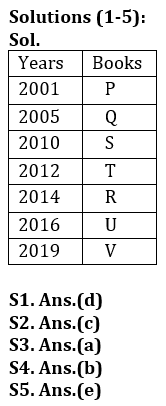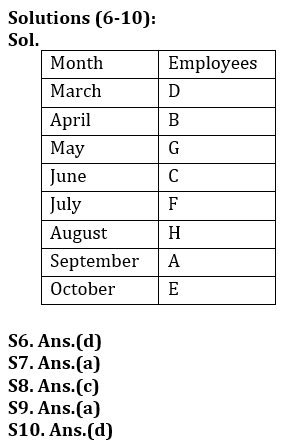Latest Banking jobs   »

# Reasoning Ability Quiz For Bank Foundation 2023-14th March

Directions (1-5) Read the following information carefully and answer the questions given below:
Seven books P, Q, R, S, T, U and V are published in same month of the different years- 2010, 2016, 2012, 2001, 2005, 2014 and 2019 but not necessarily in the same order.
Book Q published on an odd numbered year. Only three books published in between Q and U. Book R published 4 years after the year in which S published. V published on the year which is completely divisible by 3. T published on an even numbered year before U. P published one of the years before Q.

Q1. How many books published in between S and V?
(a) None
(b) Two
(c) One
(d) Three
(e) More than three

Q2. Book P published on which of the following year?
(a) 2005
(b) 2012
(c) 2001
(d) 2019
(e) 2016

Q3. Which of the following book was published before R but after S?
(a) T
(b) P
(c) Q
(d) U
(e) V

Q4. Book R published after how many years with respect to book Q published?
(a) 10 years
(b) 9 years
(c) 4 years
(d) 2 years
(e) 5 years

Q5. Which of the following book was published immediate after U published?
(a)P
(b)Q
(c)R
(d)S
(e)V

There are eight employees i.e. A, B, C, D, E, F, G and H who are born in eight different months of a same year. Months start from March to October consecutive. C is born in June. There is only one employee whose birth month is between C and B. E is born in last month of given data. H is born just before the month on which A is born but after C’s born month. F is younger than C but older than A. G is Younger than D.

Q6. Who among the following is younger than A?
(a) B
(b) D
(c) F
(d) E
(e) H

Q7. How many employees are older than F?
(a) Four
(b) Two
(c) Three
(d) Five
(e) No one

Q8. If G’s born month is interchanged with A’s born month, then G is older than which among the following employees?
(a) H
(b) B
(c) E
(d) F
(e) D

Q9. How many employees born between B and G?
(a) No one
(b) One
(c) Two
(d) Three
(e) Four

Q10. Which of the following month D is born?
(a) August
(b) June
(c) May
(d) March
(e) None of these

Direction (11-15): Study the following alphanumeric series carefully and answer the questions given below:

S * 7 B \$ * G 2 8 U 1 & A V # F 3 5 H @ 5 Y 5 M 3 2 D 8 % N 2 4

Q11. How many such numbers are there which is immediately preceded by alphabets and immediately followed by symbols?
(a) One
(b) Two
(c) Three
(d) Four
(e) None

Q12. Which of the following element is 19th from right end of the series?
(a) #
(b) F
(c) 3
(d) V
(e) None of these

Q13. How many such symbols are there which is immediately preceded by numbers and immediately followed by vowels?
(a) Two
(b) None
(c) One
(d) Four
(e) Three

Q14.How many such alphabets are there which is immediately preceded and followed by same number?
(a) Three
(b) Two
(c) One
(d) Four
(e) None

Q15. If all numbers are eliminated from the series, then, which of the following element is 11th from right end of the series?
(a) V
(b) A
(c) &
(d) #
(e) None of these

SolutionsSolutions (11-15):
S11. Ans(b)
Sol. U1&, D8%
S12. Ans(d)
S13. Ans(c)
Sol. 1&A
S14. Ans(c)
Sol. 5 Y 5
S15.Ans(b)## FAQs

### What is the selection process of the Bank Clerk?

The selection process of the Bank Clerk is Prelims & Mains.

#### Congratulations!Union Budget 2023-24: Free PDF